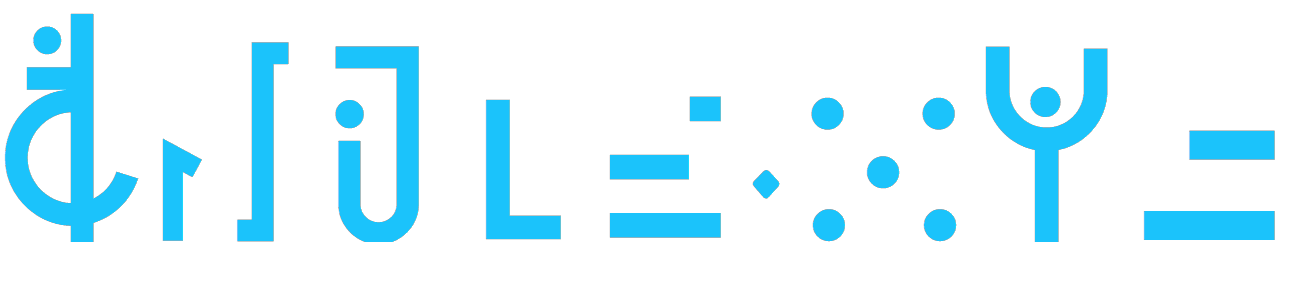## 题解 CF1178G The Awesomest Vertex

### 分块 + 斜率优化

G真的比F2清真

• 区间$a_i += x$
• 询问区间$\max{|a_i| * |b_i|}$

CF出了H没出G 菜的真实

## 有趣的矩阵乘法

（为方便，下文中“大号宝石”代指连续的$m$个分裂出来的宝石，“小号宝石”代指未分裂的单个宝石）

## 又双叒叕是题外话

$T1​$是这题。
$T2$是某次$CF\ Div1\ E$题。
$T3​$反正是某道神仙题。

## 简单递归

• 如果$[l, r]​$区间内没人，那么直接花费$A​$的代价将这段摧毁
• 如果$r > l​$（即这段区间长度$>2​$），可以选择把它切割成$\left[ l, \lfloor \frac{l + r}2\rfloor\right]​$ $\left[\lceil \frac{l + r}2\rceil, r\right]​$两段
• 如果$[l, r]$区间内有人，直接花费$b (r - l + 1)x$的代价将其摧毁。